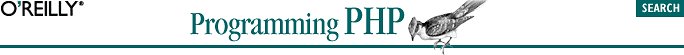home | O'Reilly's CD bookshelfs | FreeBSD | Linux | Cisco | Cisco Exam## 5.4. Multidimensional Arrays

```\$row_0 = array(1, 2, 3);
\$row_1 = array(4, 5, 6);
\$row_2 = array(7, 8, 9);
\$multi = array(\$row_0, \$row_1, \$row_2);```

You can refer to elements of multidimensional arrays by appending more []s:

`\$value = \$multi;                 // row 2, column 0. \$value = 7`
`echo("The value at row 2, column 0 is {\$multi}\n");`

Failing to use the curly braces results in output like this:

`The value at row 2, column 0 is Array`